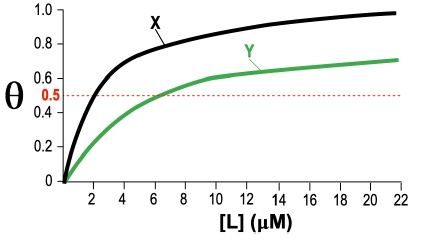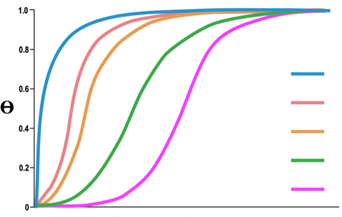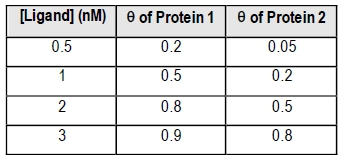Start typing, then use the up and down arrows to select an option from the list.## Biochemistry

Learn the toughest concepts covered in Biochemistry with step-by-step video tutorials and practice problems by world-class tutors

8. Protein Function

# Protein-Ligand Fractional Saturation

1
concept

## Protein-Ligand Fractional Saturation5m
Play a video:
in this video, we're going to begin our discussion on protein Ligon Fractional saturation. So first we need to define fractional saturation, which can actually be abbreviated with two different variables. The first is the Greek letter theta, and the second is the capital Letter. Why? And so some textbooks used data? Other textbooks use why, but on your exam or in some practice problems, you might find that either theta or why could be used to represent the fractional saturation. So it's important for us to be able to recognize both of these variables, however, moving forward in our course. I'm mainly going to be using data to represent the fractional saturation Onley because it's a little bit more unique and visually distinguishable. But I'll also be trying to remind you guys that every time you see Fada in our lesson, we could easily replace the theta with capital. Why? And so what exactly is this fractional saturation anyways? Well, it's just the fraction of occupied or, in other words, the fraction of saturated like in binding sites and a protein sample. And so when a ligand binding site on a protein is occupied or saturated, it just means that that, like and binding site is bound to a lie Gand. And so this fractional saturation theta or why is really just a ratio. And really, it's just the ratio of occupied proteins over the total protein content and so notice. That's exactly what we're saying down below in our image in our first box. So we can see that the fractional saturation theater, or why is really just equal to this ratio of the occupied proteins, which are just the proteins that are bound by like an over the total protein content. And so notice that that's exactly what this ratio over here is expressing is well, so the proteins that are bound by Lijun is just going to be the concentration of protein liking complex. And then the total protein is just going to be the concentration of protein liking complex, plus the concentration of free protein. Now it's also important to recognize that this expression right here for the fractional saturation actually resembles the rectangular hyperbole, a equation which recall that we covered way back when we talked about the meticulous meant in equation and so we can see that in this rectangular hyperbole equation, the access here represent the protein leg in concentration, and the B represents the concentration of free protein. And then, of course, this a right here must be equal to a value of one in our equation up above because it's not being shown. And so notice that because it resembles a rectangular hyperba that when we actually plot this data, we get a rectangular hyperbole like we see in this plot. Now, before we actually get down to this plot, it's important to note that this fractional saturation data or why can actually help us reveal the exact percentage of occupied, like and binding sites on a protein and the values of the fractional saturation theta, or why are going to range from zero at its minimum when there's absolutely no lie again, That's bound to the protein all the way up to the highest value of one when all of the protein binding sites are bound by lie game. And so at this point, we already know. So recall from our previous lesson videos that the dissociation equilibrium, constant capital, K d has units of molar ity, and it's equal to the exact lie again concentration that allows for 50% of all of the available, like in binding sites to be occupied. And so 50% corresponds with feta or the fractional saturation Equalling to a value of 0. and so down below. This plot that we see over here on the right is actually referred to as a saturation curve or ah, protein like and binding graph. And what it does is it plots the fractional saturation theta, Or why on the Y axis so we can put feta here, Or why on DSO, that's what's plotted on the Y axis and then on the X axis. Notice that it plots the lie again concentration. And so you can see that when the value of the fractional saturation theta, or why, is equal to 0.5. This is a very unique and critical point on these saturation curves or protein binding, like in binding graphs. And that's because when data is equal to 0.5, the lie again concentration that's associated with that reveals the K D, which is a measure of the affinity that the protein has for the lie again and so in our next video will be able to continue to talk more about the fractional saturation and how it applies directly to protein ligand interactions. And so I'll see you guys in that video.
2
concept

## Protein-Ligand Fractional Saturation3m
Play a video:
So now that we've introduced Protein Ligon fractional saturation in this video, we're going to focus on the maximum fada or the maximum value of the fractional saturation. And so it's important to note that for all protein ligand interactions, the equivalent of the theoretical maximum reaction velocity V max is always going to be 100% ligand binding. And so you can't have any more than 100% like and binding. And so 100% like in binding is always going to correlate with the maximum value of theta, which we know is always gonna be equal toe one. And so what we need to recall from our previous lesson videos is that the V max is actually subject to change between different enzymes. So some enzymes have a smaller V max. Other enzymes have a much, much larger V max. However, when it comes to protein ligand interactions, all protein like in interactions are always gonna have a maximum fate, a value equal to one, because you could never have mawr than 100% like in binding Now also recall from our previous lesson videos that the McHale is constant. K M is very similar to the dissociation equilibrium, constant K d. And so what this means is that the smaller the value of the K d, the stronger the proteins, affinity for that lie again. And so taking a look at our image down below, notice that we have this saturation curve here where we have the fractional saturation on the Y axis, which is either Fada or why, and notice that it ranges from zero all the way upto one and then on the X axis. What we have is the lie again concentration and notice that we have these two different curves. Here we have this black curve here for protein A and then we have this blue curve here for protein B and noticed that both protein A and protein B have the same maximum value for the theta, which is, uh, maximum value of data is one. And so this shows that regardless of what protein like an interaction that we're looking at, all protein like in interactions are gonna have a Mac state of one. And so we also need to note that, uh, the example problem here is asking which protein has a stronger affinity to the lie game. Is it protein A or is it protein B? And so what we need to recall is that the smaller the value of the k d, the stronger the proteins affinity for that lie game. And so if we're looking for a stronger affinity, we want Thio look for the smaller KD value. And so notice that this Katie value corresponding with a black herb is smaller than this Katie value corresponding with the blue curve. And so for that reason, we can say that the smaller KD belongs to protein A and so protein A therefore has a stronger affinity, so we can indicate that option. And here is the correct answer for this example problem and our next lesson video. We'll be able to talk about a different way to express the fractional saturation, so I'll see you guys in that video.
3
concept

## Protein-Ligand Fractional Saturation2m
Play a video:
So now that we know that the Max Fada value is equal toe one in this video, we're going to talk about an alternative way to express Fada. And so, through algebraic rearrangements and substitution of previous equations, Fada can also be defined in another way. And this way mathematically relates feta to the K D. And it also resembles the Mackay list meant an equation from our previous lesson videos. And so down below on the right hand side, you can see that we have the fractional saturation, which is data, or why. And we already know that it's equal to the ratio of the proteins bound by Lagan over the total concentration of protein. And so really, everything up to this point right here is review from our previous lesson video. And so here in this video, what we're saying is that this equation through algebraic rearrangements and substitution of previous equation, we can actually rearrange it to, uh, this equation right here. And this equation mathematically relates feta to the K D. So we can see we can relate theta to the value of the K. D. And it noticed that this equation here actually resembles the McHale is meant in equation from our previous lesson videos. And so notice that all we need to do is substitute the substrate concentrations with lie game concentrations substitute. The McHale is constant K m with the dissociation equilibrium, constant k d. And then, of course, the V Max. We know that the equivalent of the V max is the max data. And from our last lesson video, we know Max data is equal toe one, and so technically, here we're supposed to have Max data. But we know Max data is equal toe one. So we could just put a one here. And, of course, one times anything is just going to be that thing. And so what we can do is cross this off here because it's being multiplied by one, so it doesn't really matter. And so, really, this equation right here is the alternative way to express the fractional saturation. And so, in our next few videos will be able to get some practice utilizing all of these concepts. So I'll see you guys in our next video
4
example

## Protein-Ligand Fractional Saturation Example 15m
Play a video:
5
Problem

Consider the following graph for parts A-C.A) What is the protein-ligand dissociation constant (Kd) for protein X?

a)  2 µM.      b) 4 µM.      c) 6 µM.      d) 8 µM.

B) What is the protein-ligand dissociation constant (Kd) for protein Y?

a)  2 µM.      b) 4 µM.      c) 6 µM.     d) 8 µM.

C) Which protein has a greater affinity for ligand A?

a) Protein X.       b) Protein Y.

6
Problem

Match the dissociation constants in the table below to the appropriate curves on the graph.7
Problem

Use the table below to answer questions A, B & C below.A) Which protein has a greater affinity for their ligand?

B) According to the data in the table, what is the dissociation constant (Kd) for Protein 1?

Protein 1 Kd = ________

C. According to the data in the table, what is the association constant (K a) for Protein 2?

Protein 2 Ka = ________

8
Problem

A sample of cells has a total protein-receptor concentration of 10 mM. 25% of the protein-receptors are occupied with ligand when the concentration of free ligand is 15 mM. Calculate the Kd for the receptor-ligand interaction.